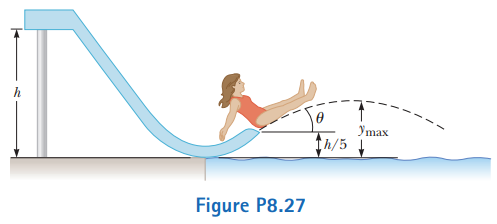# Problem: A child of mass m starts from rest and slides without friction from a height h along a slide next to a pool (Fig.  P8.27). She is launched from a height h/5 into the air over the pool. We wish to find the maximum height she reaches above the water in her projectile motion.(a) Is the child–Earth system isolated or nonisolated? Why?(b)  Is there a nonconservative force acting within the system?(c) Define the configuration of the system when the child is at the water level as having zero gravitational potential energy. Express the total energy of the system when the child is at the top of the waterslide.(d) Express the total energy of the system when the child is at the launching point.(e)  Express the total energy of the system when the child is at the highest point in her projectile motion.(f) From parts (c) and (d), determine her initial speed vi at the launch point in terms of g and h.(g) From parts (d), (e), and(f), determine her maximum airborne height ymax in terms of h and the launch angle u.(h) Would your answers be the same if the waterslide were not frictionless? Explain.

⚠️Our tutors found the solution shown to be helpful for the problem you're searching for. We don't have the exact solution yet.

###### Problem Details

A child of mass m starts from rest and slides without friction from a height h along a slide next to a pool (Fig.  P8.27). She is launched from a height h/5 into the air over the pool. We wish to find the maximum height she reaches above the water in her projectile motion.

(a) Is the child–Earth system isolated or nonisolated? Why?
(b)  Is there a nonconservative force acting within the system?
(c) Define the configuration of the system when the child is at the water level as having zero gravitational potential energy. Express the total energy of the system when the child is at the top of the waterslide.
(d) Express the total energy of the system when the child is at the launching point.
(e)  Express the total energy of the system when the child is at the highest point in her projectile motion.
(f) From parts (c) and (d), determine her initial speed vi at the launch point in terms of g and h.
(g) From parts (d), (e), and
(f), determine her maximum airborne height ymax in terms of h and the launch angle u.
(h) Would your answers be the same if the waterslide were not frictionless? Explain.